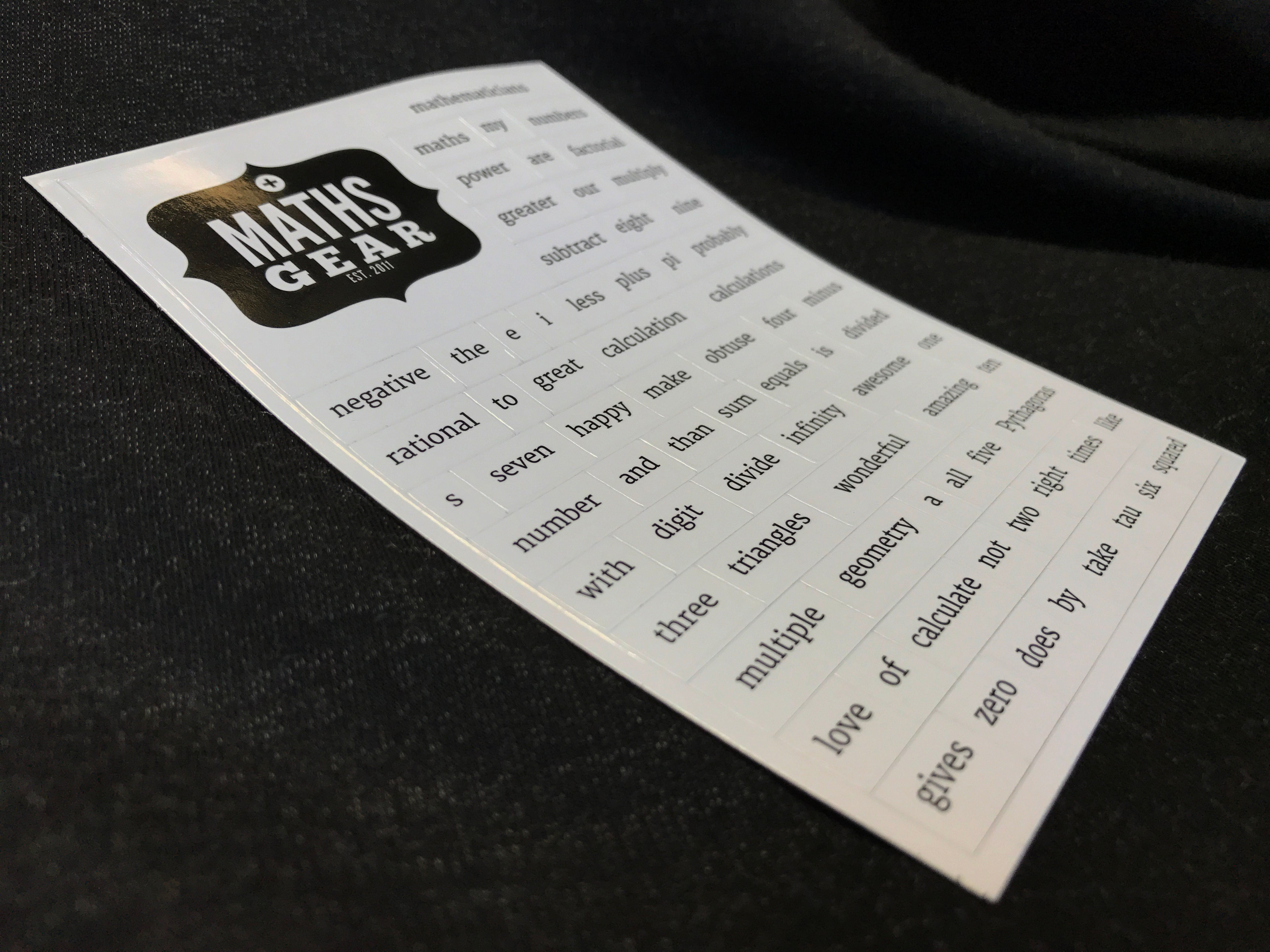1# Maths Gear Magnetic Poetry Set

## £4.49

These mathematical magnets can be arranged to form poetry. They can also just be arranged to form true mathematical facts, but we’d argue that all maths is poetry. So it’s the same thing. For example:

all our triangles take
the sum of three
and with awesome multiple geometry
mathematicians make
greater than one eighth e

And

eight subtract nine equals negative zero factorial

See. Indistinguishable.

Fun fact: Originally we thought we’d write the whole blurb about the maths magnets using only the maths magnets themselves. But this is far as we got:

i make amazing maths with my digits

Price = p87 pence = £4.49